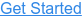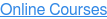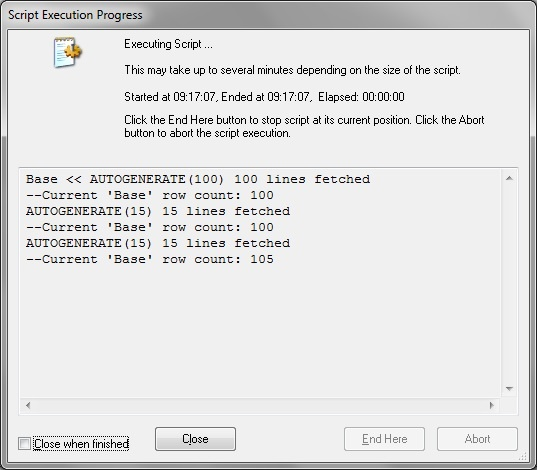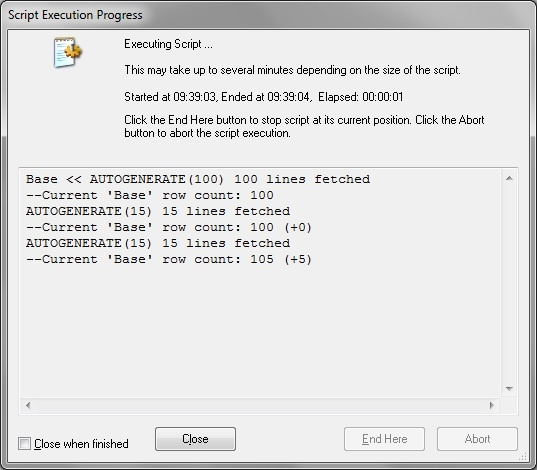Where do you want to go?# Logging row counts

by Mike Steedle, on Apr 27, 2013 9:44:54 AM

Something that would be nice to see in QVW log files is the number of rows that result from executing a join.

It would be useful to test assumptions about unfamiliar data, like the distinctness of a field on which you are joining, and for people who are new to transforming data and trying to understand the impacts of different join types. As it is, the "lines fetched" that appear in the log after the join is the number of rows retrieved in the load that was joined to another table.

To help remedy this, I wrote a subroutine that can be called to return the row count of any table at any point during the script execution:

```Sub RowCount(vTableName)
LET vRowCount = Num(NoOfRows('\$(vTableName)'),'#,##0');
TRACE --Current '\$(vTableName)' row count: \$(vRowCount);
End Sub```

Here's how you call it, where "Base" is the table name:

`CALL RowCount ('Base')`

And here is the result in the execution window, which is mirrored in the log file:Here is a slightly more advanced version of the subroutine, which displays the delta, as well, if the same table is called multiple times in a row, which would also be nice to use following concatenate loads:

```LET vLastTableName = ;
Sub RowCount(vTableName)
LET vRowCount = Num(NoOfRows('\$(vTableName)'),'#,##0');
LET vRowCountChange = If(vTableName = vLastTableName, ' (' & Num(vRowCount-vLastRowCount,'+#,##0','-#,##0') & ')', '');
TRACE --Current '\$(vTableName)' row count: \$(vRowCount)\$(vRowCountChange);
LET vLastTableName = vTableName;
LET vLastRowCount = vRowCount;
End Sub```